### GATE 2000 ECE Video Solutions on Analog Circuits (Analog Electronics)

1. In the differential amplifier of the figure, if the source resistance of the current source IEE is infinite, then the common mode gain isa. Zero
b. Infinite
c. Indeterminate
d. (Vin1 + Vin2) / 2VT

2. In the circuit shown in figure, the output voltage Vo isa. – 1 volts
b. 2 volts
c. + 1 volts
d. + 15 volts

3. Introducing a resistor in the emitter of a CE amplifier stabilizes the dc operating point against variations in
a. Only the temperature
b. Only the β of the transistor
c. Both temperature and β
d. None of the above

4. The current gain of a bipolar transistor drops at high frequencies because of
a. Transistor capacitances
b. High current effects in the base
c. Parasitic inductance effects
d. The Early Effect

5. If the OP-AMP in the figure is ideal, then the output voltage Vo is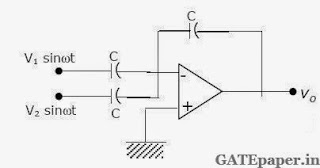a. Zero
b. (V1 – V2) sinωt
c. – (V1 + V2) sinωt
d. (V1 + V2) sinωt

6. The configuration of the figure is aa. Precision rectifier
b. Hartley oscillator
c. Butterworth high pass filter
d. Wien-bridge oscillator

7. Assume that the OP-AMP of the figure is ideal. If Vi is a triangular wave, then Vo will bea. Square wave
b. Triangular wave
c. Parabolic wave
d. Sine wave

8. The most commonly used amplifier in Sample and Hold circuits is
a. Unity gain inverting amplifier
b. Unity gain non-inverting amplifier
c. An inverting amplifier with a gain of 10
d. An inverting amplifier with a gain of 100

9. If the OP-AMP in the figure has an input offset voltage of 5 mV and an open loop voltage gain of 10,000. Then the output Vo will be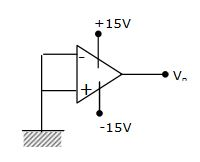a. 0 volts
b. 5 mV
c. + 15 volts or – 15 volts
d. + 50 volts or – 50 volts

10. a. For the circuit shown, plot Vo under steady state conditions, with and without capacitor C. Assume that the diode is ideal.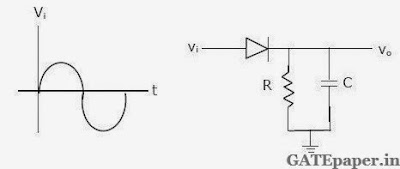b. Design a circuit using two ideal diodes, one resistor and two voltage sources that would convert the input voltage to the output voltage as shown in figure. The resistor value need not be specified.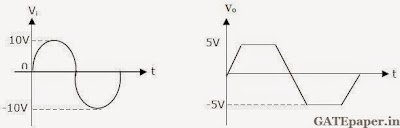11. For the amplifier circuit shown, IC = 1.3 mA, RC = 2 kΩ, RE = 500 Ω, VT = 26 mV, β = 100, VCC = 15 volts, VS = 0.01 sin(ωt) volts and Cb = Ce = 10 µF.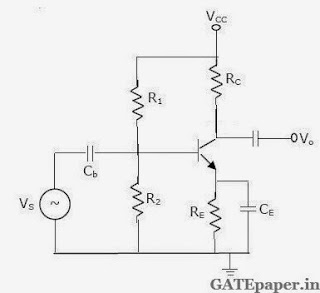a. What is the small signal voltage gain, Vo/VS
b. What is the approximate voltage gain if Ce is removed?
c. What will be the output Vo, if Cb is short circuited?

12. For a feedback amplifier, the open loop transfer function has three poles at 100 k rad/sec, 1 M rad/sec and 10 M rad/sec. The low frequency open loop gain is 1000 and the feedback factor (β) is 1. Use Bode plots to determine the phase margin of the amplifier. Is the amplifier stable?

Solution: Amplifier is Unstable.

13. Below figure shown is a common base amplifier.a. Write expressions for the time constants associated with the capacitances Cb and Cs.
b. What is the approximate lower cutoff frequency of the amplifier?

### GATE 2001 ECE Video Solutions on Analog Circuits (Analog Electronics)

1. The current gain of a BJT is
a. gmro
b. gm / ro
c. gmrπ
d. gm / rπ

2. The ideal OP-AMP has the following characteristics
a. Ri = ∞, AV= ∞, Ro = 0
b. Ri = 0, AV= ∞, Ro = 0
c. Ri = ∞, AV= ∞, Ro = ∞
d. Ri = 0, AV= ∞, Ro = ∞

3. Consider the following two statements :
Statement 1: Astable Multivibrator can be used for generating square wave.
Statement 2: Bistable Multivibrator can be used for storing binary information.
a. Only statement 1 is correct.
b. Only statement 2 is correct.
c. Both the statements 1 and 2 are correct.
d. Both the statements 1 and 2 are incorrect.

4. An NPN BJT has gm = 38 mA/volt, Cµ = 10-14 F, Cπ = 4x10-13 F and DC current gain β = 90. For this transistor fτ and fβ are
a. fτ = 1.64 x 108 Hz and fβ = 1.47 x 1010 Hz
b. fτ = 1.47 x 1010 Hz and fβ = 1.64 x 108 Hz
c. fτ = 1.33 x 1012 Hz and fβ = 1.47 x 1010 Hz
d. fτ = 1.47 x 1010 Hz and fβ = 1.33 x 1012 Hz

5. The following circuit shown in the figure is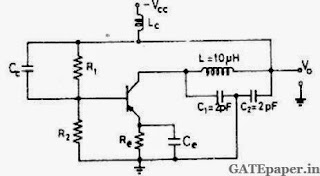a. Hartley oscillator with fosc = 79.6MHz
b. Colpitts oscillator with fosc = 50.3MHz
c. Hartley oscillator with fosc = 159.2MHz
d. Colpitts oscillator with fosc = 159.2MHz

6. The inverting OP-AMP shown in the figure has an open loop gain of 100. The closed loop gain Vo/Vsis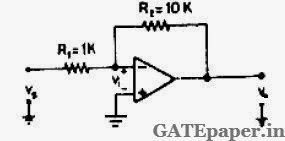a. – 8
b. – 9
c. – 10
d. – 11

7. In the figure, assume the OP-AMPs to be ideal. The output Vo of the circuit is8. An emitter follower amplifier is shown below, where Zi is the impedance looking into the base of the transistor and Zo is the impedance looking into the emitter of the transistor.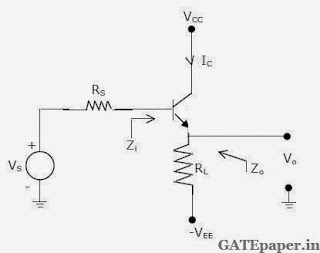a. Draw the small signal equivalent circuit of the amplifier.
b. Obtain an expression for Zi.
c. Obtain an expression for Zo.
d. Determine Zi and Zo, if a capacitor C is connected across R1.

9. In the circuit shown, assume that the OP-AMP is ideal.a. Obtain an expression for Vo in terms of Vs, R and the reverse saturation current Is of the transistor.
b. If R = 1 Ω, Is = 1 pA and the thermal voltage VT = 25 mV, then what is the value of the output voltage Vo for an input voltage Vs of 1 volt?
c. Suppose that the transistor in the feedback path is replaced by a PN junction diode with a reverse saturation current of Is. The P-side of the diode is connected to node A and the N-side to node B. then what is the expression for Vo in terms of Vs, R and Is ?

### GATE 2002 ECE Video Solutions on Analog Circuits (Analog Electronics)

1. In a negative feedback amplifier using voltage-series (Voltage sampling and series mixing) feedback,
a. Ri decreases and Ro decreases
b. Ri decreases and Ro increases
c. Ri increases and Ro decreases
d. Ri increases and Ro increases

2. A 741 OP-AMP has a gain-bandwidth product of 1 MHz. A non-inverting amplifier using this OP-AMP and having a voltage gain of 20 dB will exhibit a –3 dB bandwidth of
a. 50 kHz
b. 100 kHz
c. 100/17 kHz
d. 1000/7.07 kHz

3. Three identical RC coupled transistor amplifiers are cascaded. If each of the amplifiers has a frequency response as shown in the figure, the overall frequency response is as given in4. The circuit in the figure employs positive feedback and is intended to generate sinusoidal oscillations. If at a frequency fo, β(f) = Vf(f)/Vo(f) = 1/6 ∟0o, then to sustain oscillations at this frequencya. R2 = 6R1
b. R2 = 5R1
c. R2 = R1/6
d. R2 = R1/5

5. An amplifier using an OP-AMP with a slew rate SR = 1 V/µsec has a gain of 40 dB. If this amplifier has to faithfully amplify sinusoidal signals form dc to 20 kHz without any slew rate induced distortion, then the input signal level must not exceed
a. 795 mV
b. 395 mV
c. 79.5 mV
d. 39.5 mV

6. The voltage gain Av = Vo/Vi of the JFET amplifier shown in the figure is IDSS = 10 mA, VP = 5 volts. Assume C1, C2 and Cs to be very large.a. + 16
b. – 16
c. + 8
d. – 6

7. A triangular voltage waveform Vi(t) is applied at the input to the circuit shown. Assume the diodes to be ideal.a. Determine the output Vo(t)
b. Neatly sketch the output waveform superimposed on the input Vi(t) and label the key points.

8. Below figure shown is a 2 – stage amplifier.The transistors Q1 and Q2 are identical with current gain β = 100, further βDC = βac = β. The zener diode Dz has a break down region and its dynamic resistance rz is zero. The capacitors C1 and C2 are large and provide negligible impedance at signal frequencies.
a. Identify the configuration in each of the amplifier stages. (i.e. whether CE, CC or CB)
b. Determine the quiescent quantities of IC1 and VC1.
c. Determine an expression for the voltage gain Vo/Vs and determine its value. (Assume VBE = 0.7 volts, ro = ∞ and thermal voltage VT = 25mV)
Solution:

9. Consider the circuit shown. Assume the operational amplifier is ideal.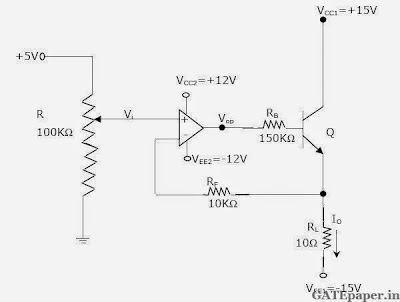a. In which mode is the BJT operating (active or saturation or cutoff)? Justify your answer.
b. Obtain an expression relating the output current Io and the input voltage Vi.
c. Determine Io and Vop if Vi = 2 volt. Assume β = 99 and VBE = 0.7 volts.

### GATE 2003 ECE Video Solutions on Analog Circuits (Analog Electronics)

1. Choose the correct match of input resistance of various amplifier configurations shown below:2. The circuit shown in the figure is best described as a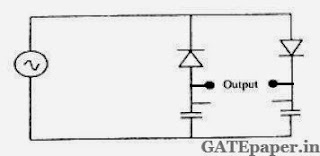a. Bridge rectifier
b. Ring modulator
c. Frequency discriminator
d. Voltage doubler

3. If the input to the ideal comparator shown in the figure is a sinusoidal signal of 8 volts peak to peak, without any DC component, then the output of the comparator has a duty cycle ofa. 1/2
b. 1/3
c. 1/6
d. 1/2

4. If the differential voltage gain and the common mode voltage gain of a differential amplifier are 48 dB and 2 dB respectively, then common mode rejection ratio is
a. 23 dB
b. 25 dB
c. 46 dB
d. 50 dB

5. Generally, the gain of a transistor amplifier falls at high frequencies due to the
a. Internal capacitance of the device
b. Coupling capacitor at the input
c. Skin effect
d. Coupling capacitor at the output

6. An amplifier without feedback has a voltage gain of 50, input resistance of 1 KΩ and output resistance of 2.5 KΩ. The input resistance of the current-shunt negative feedback amplifier using the above amplifier with a feedback factor of 0.2 is
a. 1/11 KΩ
b. 1/5 KΩ
c. 5 KΩ
d. 11 KΩ

7. In the amplifier circuit shown in the figure, the values of R1 and R2 are such that the transistor is operating at VCE = 3 volts and IC = 1.5 mA, when its β is 150.for a transistor with β of 200, the operating point (VCE, IC) isa. (2 volts, 2 mA)
b. (3 volts, 2 mA)
c. (4 volts, 2 mA)
d. (4 volts, 1 mA)

8. The oscillator circuit shown in the figure has an ideal inverting amplifier. Its frequency of oscillation in Hertz is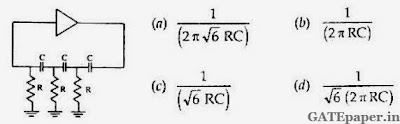9. The output voltage of the regulated power supply shown in the figure is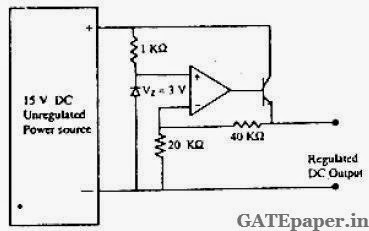a. 3 volts
b. 6 volts
c. 9 volts
d. 12 volts

10. If the OP-AMP in the figure is ideal, the output voltage VOUT will be equal to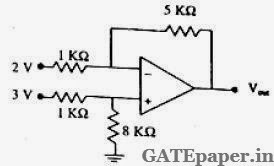a. 1 volt
b. 6 volts
c. 14 volts
d. 17 volts

11. Three identical amplifiers with each one having a voltage gain of 50, input resistance of 1 KΩ and output resistance of 250 Ω are cascaded. The open circuit voltage gain of the combined amplifier is
a. 49 dB
b. 51 dB
c. 98 dB
d. 102 dB

12. An ideal saw-tooth voltages waveform of frequency of 500 Hz and amplitude 3 volts is generated by charging a capacitor of 2 µF in every cycle. The charging requires
a. Constant voltage source of 3 volts for 1 ms
b. Constant voltage source of 3 volts for 2 ms
c. Constant current source of 1 mA for 1 ms
d. Constant current source of 3 mA for 2 ms

### GATE 2004 ECE Video Solutions on Analog Circuits (Analog Electronics)

1. An ideal OP-AMP is an ideal
a. Voltage Controlled Current Source
b. Voltage Controlled Voltage Source
c. Current Controlled Current Source
d. Current Controlled Voltage Source

2. Voltage series feedback (also called series-shunt feedback) results in
a. Increase in both input and output impedances
b. Decrease in both input and output impedances
c. Increase in input impedance and decrease in output impedance
d. Decrease in input impedance and increase in output impedance

3. The circuit in the figure is aa. Low pass filter
b. High pass filter
c. Band pass filter
d. Band reject filter

4. A bipolar transistor is operating in the active region with a collector current of 1 mA. Assuming that the β of the transistor is 100 and the thermal voltage (VT) is 25 mV. The Transconductance (gm) and the input resistance (rπ) of the transistor in the common emitter configuration are
a. gm = 25 mA/V and rπ = 15.625 KΩ
b. gm = 40 mA/V and rπ = 4.0 KΩ
c. gm = 25 mA/V and rπ = 2.5 KΩ
d. gm = 40 mA/V and rπ = 2.5 KΩ

5. the value of capacitor C required for sinusoidal oscillations of frequency 1 kHz in the circuit of the figure is6. in the OP-AMP circuit given in the figure, the load current iL is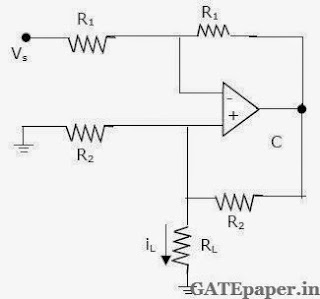a. – VS / R2
b. VS / R2
c. – VS / RL
d. VS / R1

7. In the full wave rectifier using two ideal diodes, Vdc and Vm are the dc and peak values of the voltage respectively across a resistive load. If PIV is the peak inverse voltage of the diode, then the appropriate relationships for this rectifier are### GATE 2005 ECE Video Solutions on Analog Circuits (Analog Electronics)

1. The effect of current shunt feedback in an amplifier is to
a. Increase the input resistance and decrease the output resistance.
b. Increase both input and output resistances
c. Decrease both input and output resistances
d. Decreases the input resistance and increase the output resistance

2. The input resistance Ri of the amplifier shown in figure isa. 30/4 KΩ
b. 10 KΩ
c. 40 KΩ
d. Infinite

3. The cascode amplifier is a multistage configuration of
a. CC – CB
b. CE – CB
c. CB – CC
d. CE – CC

4. A square pulse of 3 volts amplitude is applied to RC circuit shown in figure. The capacitor is initially uncharged. The output voltage Vo at time t = 2 sec isa. 3 volts
b.– 3 volts
c. 4 volts
d. – 4 volts
Solution:

5. For an NPN transistor connected as shown in figure, VBE = 0.7 volts. Given that reverse saturation current of the junction at room temperature 300oK is 10-13 amp, the emitter current isa. 30 mA
b. 39 mA
c. 49 mA
d. 20 mA

6. The voltage eo indicated in figure has been measured by an ideal voltmeter, which of the following can be calculated?a. Bias current of the inverting input only
b. Bias current of the inverting and non-inverting inputs only
c. Input offset current only
d. Both the bias currents and the input offset current.

7. The OP-AMP circuit shown in figure is a filter. The type of filter and its cut-off frequency are respectively.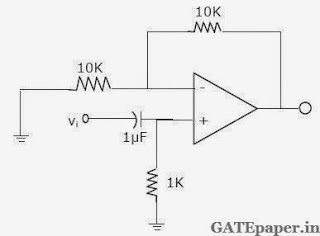8. In the differential amplifier shown in figure, a large value of REa. Increases both the differential and common mode gains
b. Increases the common mode gain only
c. Decreases the differential mode gain only
d. Decreases the common mode gain only

9. The circuit using a BJT with β = 50 and VBE = 0.7 volts is shown in figure. The base current IB and the collector voltage VC are respectively.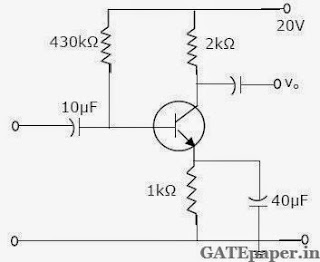a. 43 µA and 11.4 volts
b. 40 µA and 16 volts
c. 45 µA and 11 volts
d. 50 µA and 10 volts

10. Given the ideal operational amplifier circuit shown in figure indicate the correct transfer characteristics assuming ideal diodes with zero cut-in voltage.Common Data Questions for 11, 12 and 13:
Given rd = 20 KΩ, IDSS = 10 mA, VP = - 8 volts.11. Zi and Zo of the circuit are respectively
a. 2 MΩ and 2 KΩ
b. 2 MΩ and 20/11 KΩ
c. Infinity and 2 KΩ
d. Infinity and 20/11 KΩ

12. ID and VDS under DC conditions are respectively
a. 5.625 mA and 8.75 Volts
b. 7.50 mA and 5.0 Volts
c. 4.50 mA and 11.0 Volts
d. 6.25 mA and 7.5 Volts

13. Transconductance in milli-siemens (mS) and the voltage gain of the amplifier are
a. 1.875 mS and 3.41
b. 1.875 mS and – 3.41
c. 3.30 mS and – 6
d. 3.30 mS and 6

### GATE 2006 ECE Video Solutions on Analog Circuits (Analog Electronics)

1. The input impedance(Zi) and output impedance (Zo) of an ideal Transconductance (voltage controlled current source) amplifier are
a. Zi = 0, Zo = 0
b. Zi = 0, Zo = ∞
c. Zi = ∞, Zo = 0
d. Zi = ∞, Zo = ∞

2. For the circuit shown in the following figure, the capacitor C is initially uncharged. At t = 0, the switch S is closed. The voltage VC across the capacitor at t = 1 ms is _____. Assume the OP – AMP is supplied with ± 15 volts.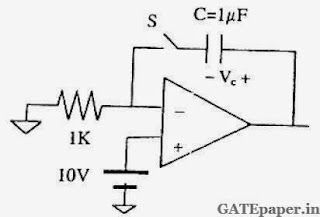a. 0 volts
b. 6.3 volts
c. 9.45 volts
d. 10 volts

Common Data for Questions (3, 4 and 5):
In the transistor amplifier circuit shown in the figure below, the transistor has the following parameters: βDC = 60, VBE = 0.7 volts, hie -> ∞, hoe -> ∞. The capacitance CC can be assumed to be infinite.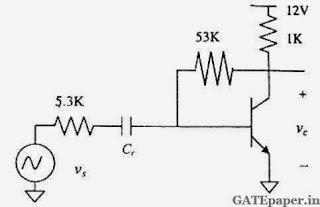3. Under the DC conditions, the collector to emitter voltage drop is
a. 4.8 volts
b. 5.3 volts
c. 6.0 volts
d. 6.6 volts

4. If βDC is increased by 10%, the collector to emitter voltage drop
a. Increases by less than or equal to 10%
b. Decreases by less than or equal to 10%
c. Increases by more than 10%
d. Decreases by more than 10%

5. The small signal gain of the amplifier Vo/Vs is
a. – 10
b. – 5.3
c. + 5.3
d. + 10

A regulated power supply shown in the figure below has an unregulated input of 15 volts and generates a regulated output Vout. Use the component values shown in the figure.6. The power dissipation across the transistor Q1 shown in the figure is
a. 4.8 volts
b. 5.0 volts
c. 5.4 volts
d. 6.0 volts

7. If the unregulated voltage increases by 20%, then power dissipation across the transistor Q1
a. Increases by 20%
b. Increases by 50%
c. Remains unchanged
d. Decreases by 20%

### GATE 2007 ECE Video Solutions on Analog Circuits (Analog Electronics)

1. In a Transconductance amplifier, it is desirable to have
a. A large input resistance and a large output resistance
b. A large input resistance and a small output resistance
c. A small input resistance and a large output resistance
d. A small input resistance and a small output resistance

2. For the OP-AMP circuit shown in the figure, Vo isa. – 2 volts
b. – 1 volts
c. – 0.5 volts
d. + 0.5 volts

3. In the OP-AMP circuit shown, assume that the diode current follows the equation I = ISexp(V/VT). For Vi = 2 Volts, Vo = V01, and for Vi = 4 Volts, Vo = V02. The relationship between V01 and V02 isa. V02 = √2 V01
b. V02 = e2 V01
c. V02 = V01 ln2
d. V02 - V01 = VT ln2

Consider the OP-AMP circuit shown in the figure.4. The Transfer function Vo(s)/ Vi(s) is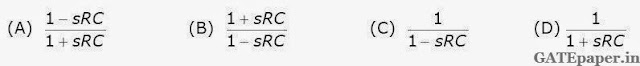5. If Vi = V1 sin(ωt) and Vo = V2 sin(ωt+ø), then the minimum and maximum values of ø (in radians) are respectively
a. – π/2 and π/2
b. 0 and π/2
c. – π and 0
d. – π/2 and 0